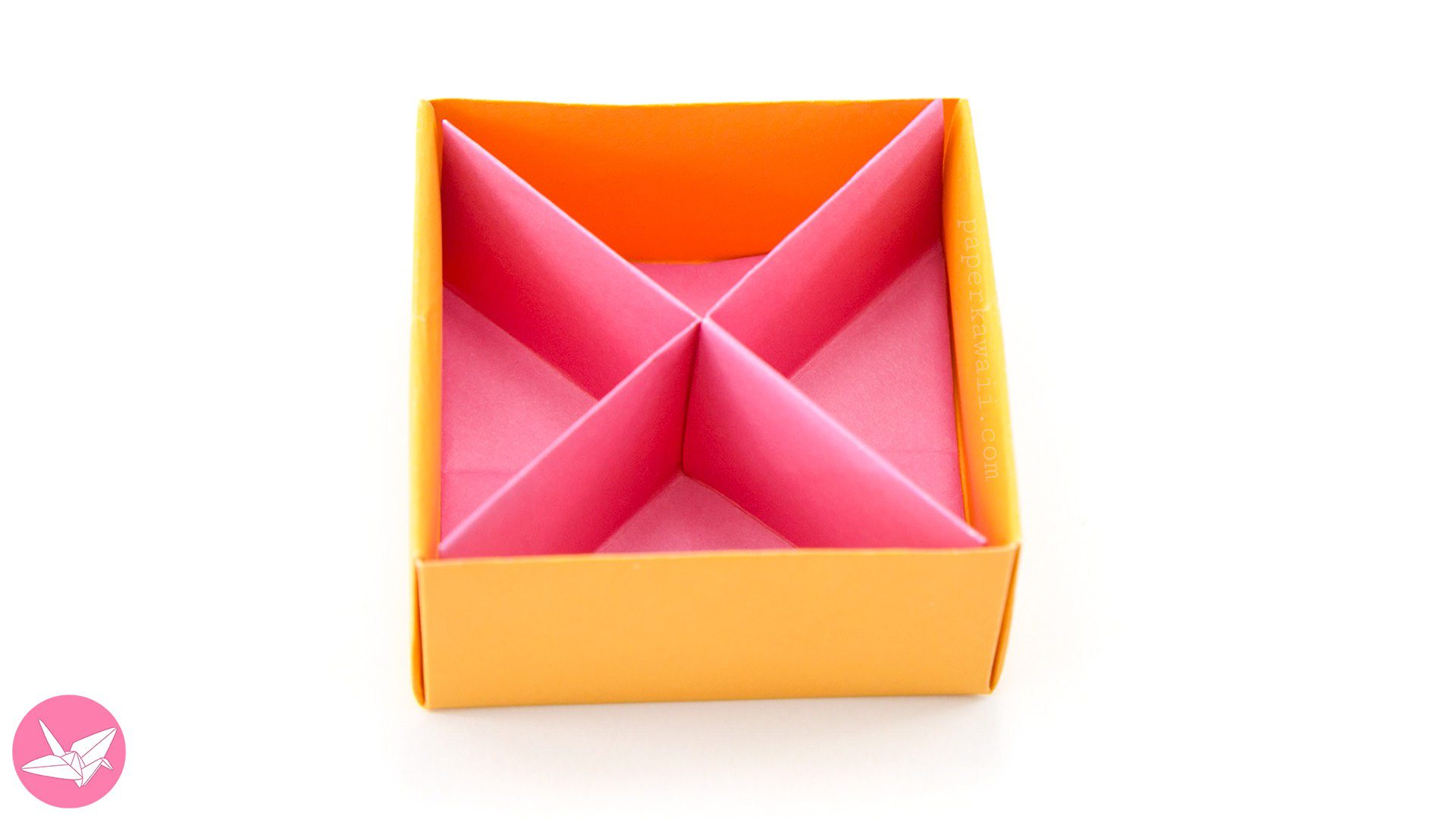This useful origami box divider is diagonal like an X, instead of squares you get triangular sections. Great to keep jewellery gifts (& other items) separated.

As requested, here is a tutorial for my diagonal origami box divider. It took a while to get this divider working properly.

For this divider, the sections are triangular. The folds for this divider might seem a little strange, that’s because I wanted people to be able to start with the same sized paper as the masu box, not some strange measurement, so with this folding sequence you can start with the same size, just trim 1cm off the size (not perfect I know).

Even though these diagonal divisions might not be as practical as the original square sections divider, they are very appealing.

Tutorial for the lid pictured above can be found here.

Level: ★★✮☆☆

### You will need:

▸ 1 sheet of square paper. (I recommend thinner paper)

So for example if you used 15 x 15 cm for the masu box, you’d need to use 14 x 14 cm for this divider.

(You may need to alter this depending on how thick your paper is.)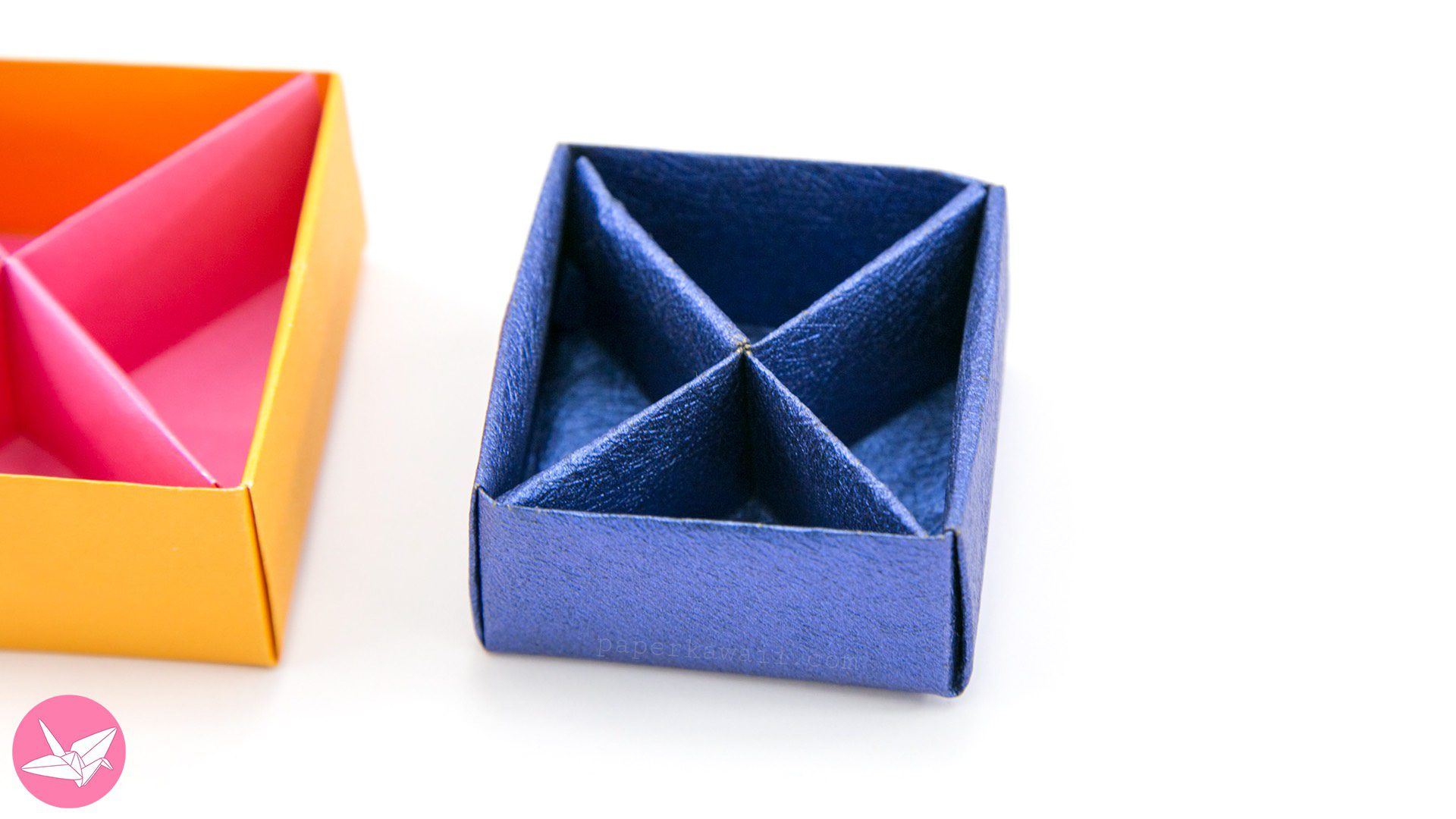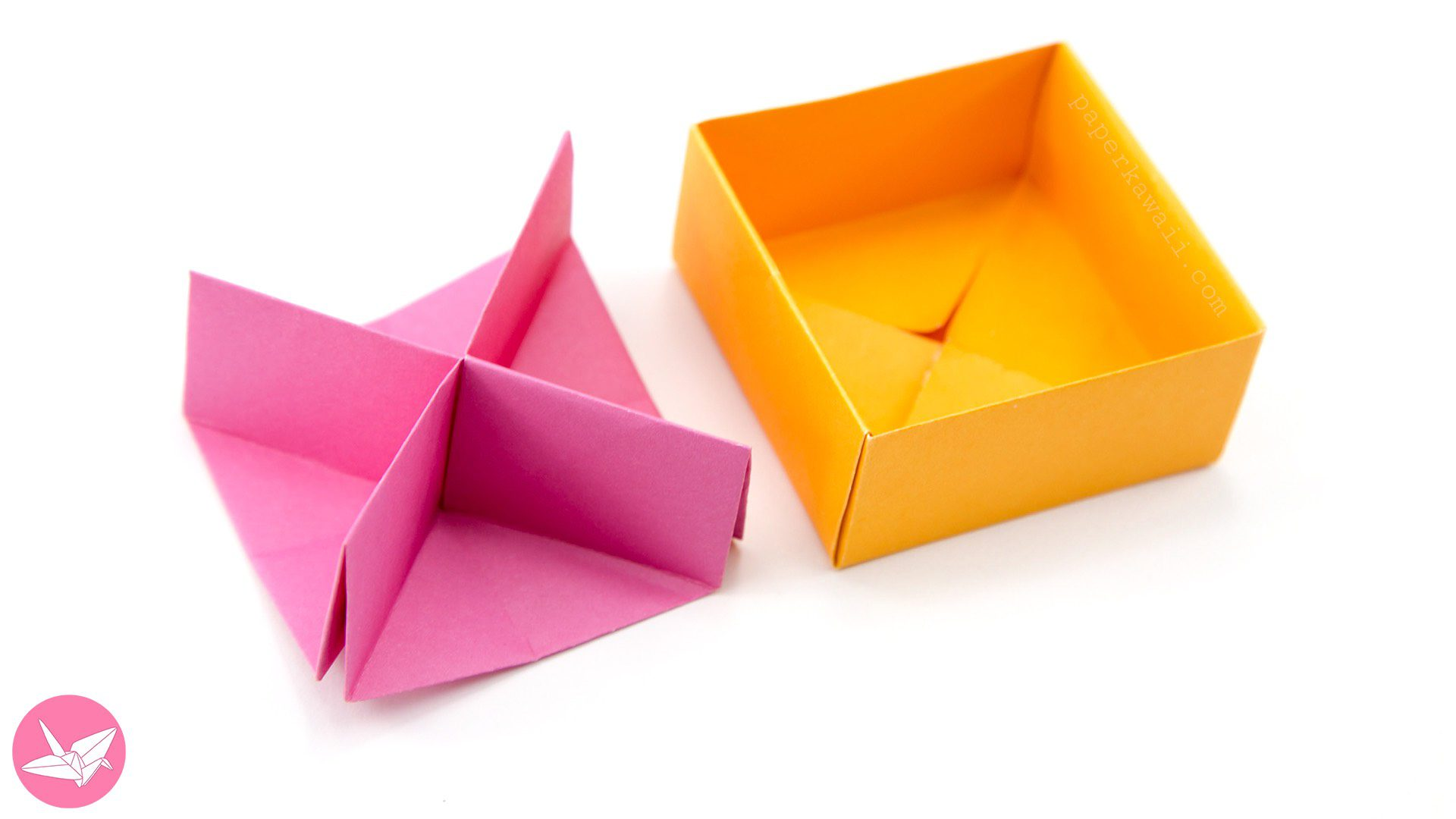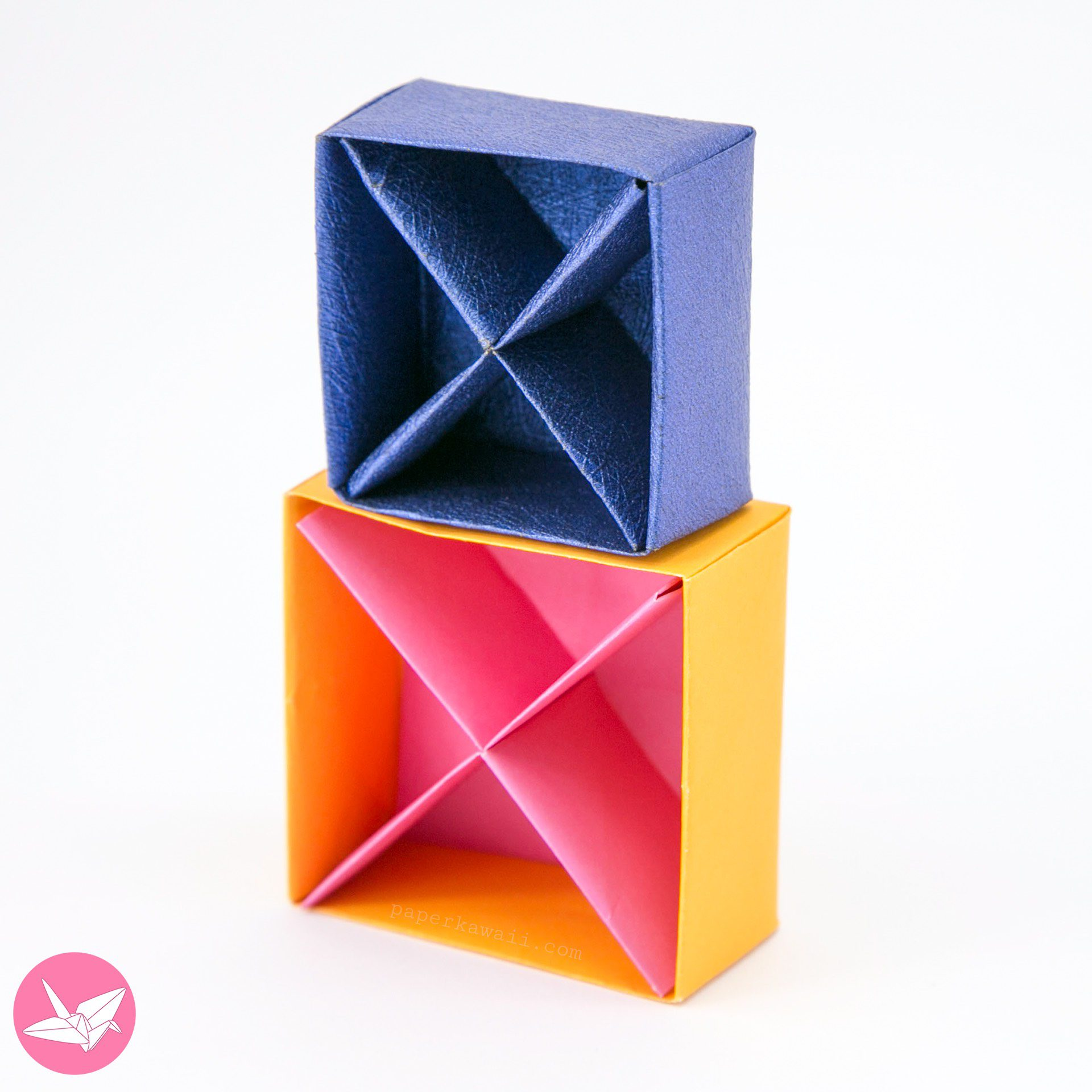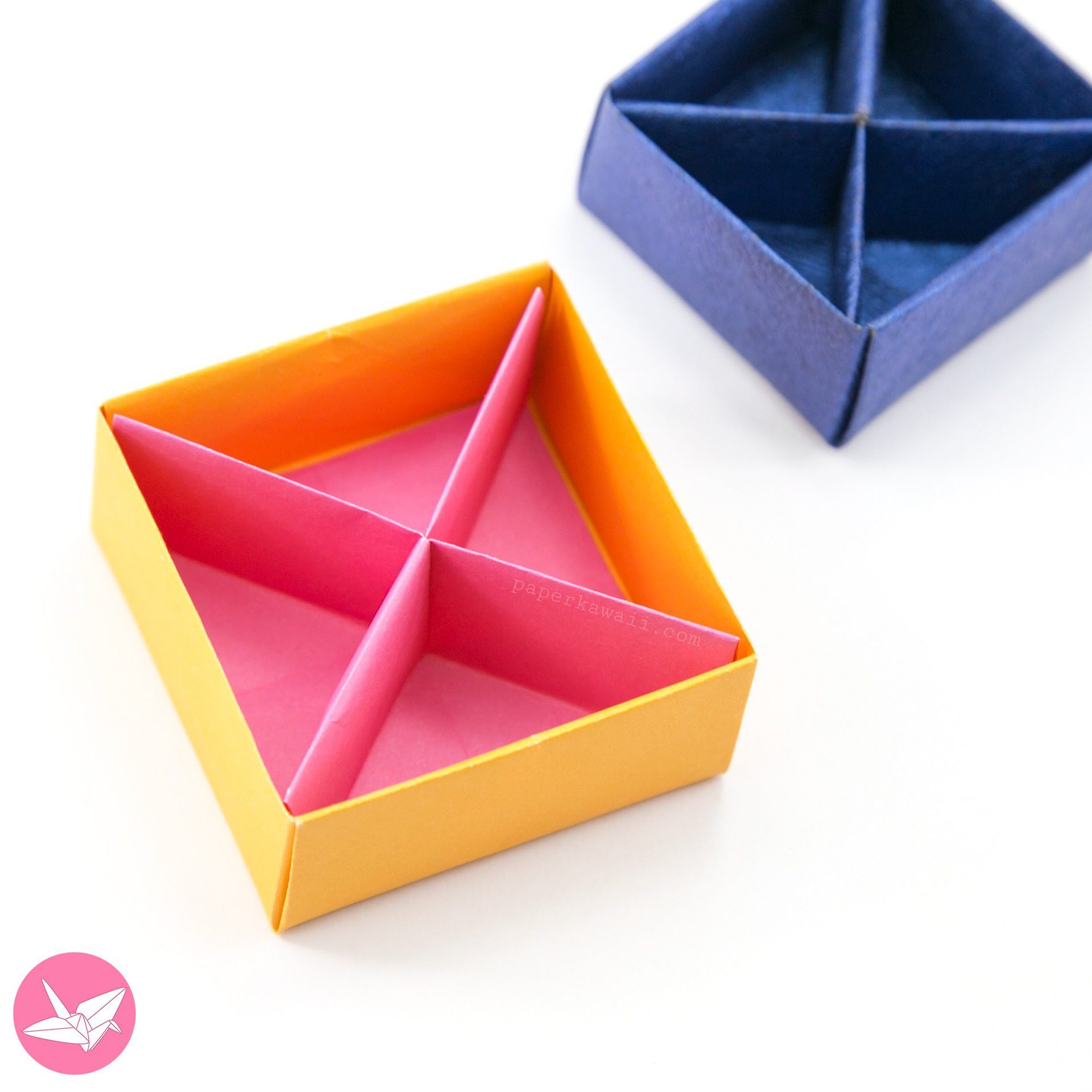## Use this origami divider in these origami projects:Origami Desk Organiser (tutorial here)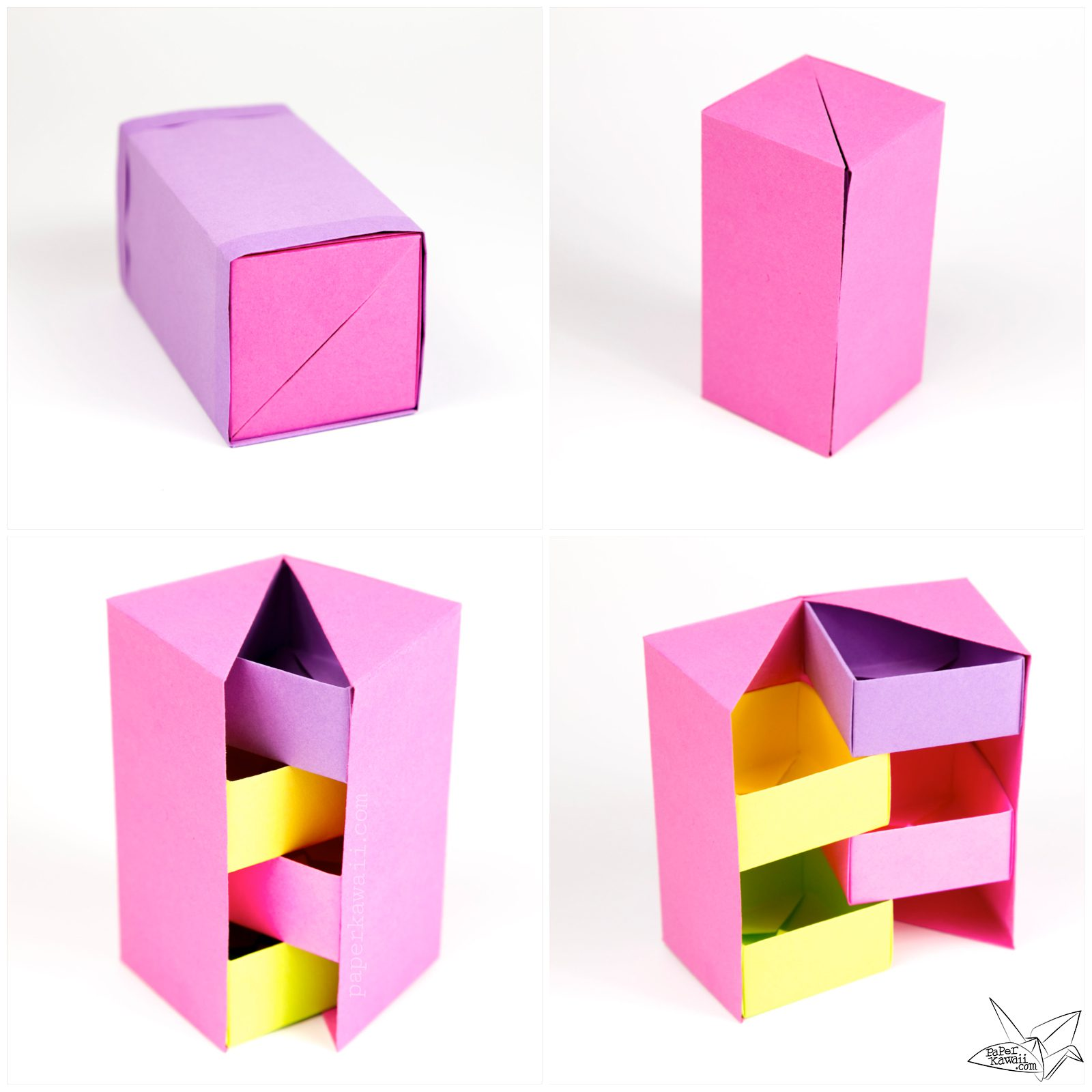Origami Secret Stepper Box (tutorial here)